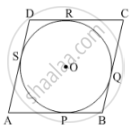Share

Books Shortlist

Prove that a Parallelogram Circumscribing a Circle is a Rhombus. - CBSE Class 10 - Mathematics

ConceptNumber of Tangents from a Point on a Circle

Question

Prove that a parallelogram circumscribing a circle is a rhombus.

Solution

Given: ABCD be a parallelogram circumscribing a circle with centre O.

To prove: ABCD is a rhombus.We know that the tangents drawn to a circle from an exterior point are equal in length.

∴ AP = AS, BP = BQ, CR = CQ and DR = DS.

AP + BP + CR + DR = AS + BQ + CQ + DS

(AP + BP) + (CR + DR) = (AS + DS) + (BQ + CQ)

∴ AB + CD = AD + BC or 2AB = 2BC                     (since AB = DC and AD = BC)

∴ AB = BC = DC = AD.

Therefore, ABCD is a rhombus.

Hence, proved.

Is there an error in this question or solution?

Video TutorialsVIEW ALL 

Solution Prove that a Parallelogram Circumscribing a Circle is a Rhombus. Concept: Number of Tangents from a Point on a Circle.
S